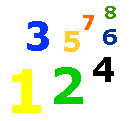# Division Assessment

Division Lesson & Worksheet - My Schoolhouse - Online Learning

Complete the following division problems.

 9 -:- 4 = remainder 1 13 -:- 4 = 3 remainder23 -:- 7 = 3 remainder 11 -:- 3 = remainder 228 -:- 5 = 5 remainder 47 -:- 7 = remainder 555 -:- 9 = 6 r 65 -:- 7 = r 2

Complete the following division problems.

 18 -:- 5 = 3 r 23 -:- 5 = r 3 33 -:- 6 = 5 r51 -:- 7 = r 2 24 -:- 5 = r 4 32 -:- 7 = 4 r44 -:- 9 = r 26 -:- 4 = r 76 -:- 8 = r58 -:- 6 = r 40 -:- 6 = r 88 -:- 9 = r

Complete the short division problems below.

 9 -:- 3 = 390 -:- 3 = 900 -:- 3 = 300 12 -:- 4 = 120 -:- 4 = 30 1,200 -:- 4 =20 -:- 4 = 5200 -:- 4 = 2,000 -:- 4 = 500 18 -:- 3 = 6180 -:- 3 = 1,800 -:- 3 =27 -:- 9 = 3270 -:- 9 = 2,700 -:- 9 = 48 -:- 8 = 480 -:- 8 = 4,800 -:- 8 =63 -:- 7 = 630 -:- 7 = 6,300 -:- 7 = 72 -:- 9 = 720 -:- 9 = 7,200 -:- 9 =560 -:- 8 = 705,600 -:- 8 = 56,000 -:- 8 = 540 -:- 6 = 5,400 -:- 6 = 54,000 -:- 6 =

Complete the following long division problems.

 r 0 2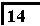-14 0

 r 0 4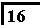-16 0

 r 1 5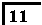-10 1r 3 4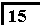-12 3

 r 5-15 2

 r 6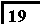-18 1r 5-20

 r 1 5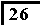-25

 r 2 9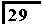- 2r 1 5-30 1

 r 7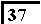-35

 r 8-40r 3 7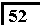- 3

 r 2 7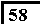-56

 r 2 7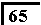- 2r 3 8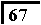- 3

 r 9-72

 r 2 9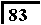- 2

 Thank You for using My Schoolhouse